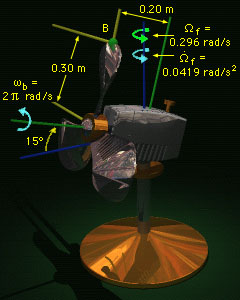Ch 9. 3-D Motion of a Rigid Body Multimedia Engineering Dynamics Translating Coordinates Rotating Coordinates Inertia & Ang. Momentum Equationsof Motion
 Chapter - Particle - 1. General Motion 2. Force & Accel. 3. Energy 4. Momentum - Rigid Body - 5. General Motion 6. Force & Accel. 7. Energy 8. Momentum 9. 3-D Motion 10. Vibrations Appendix Basic Math Units Basic Equations Sections Search eBooks Dynamics Fluids Math Mechanics Statics Thermodynamics Author(s): Kurt Gramoll ©Kurt GramollDYNAMICS - CASE STUDY IntroductionProblem Diagram An oscillating fan is rotating about a vertical axis. An unsuspecting fly flies into the fan and hits the tip of one of the blades when the blade is in the vertical position. What will happen to the fly? What is known: At the instant the fly hits the fan, the fan is rotating about the vertical axis with an angular speed of Ωf = 0.296 rad/s, increasing at a rate of dΩf/dt = 0.0419 rad/s2. The blades of the fan are spinning at a constant rate of ωb = 2π rad/s. The fan has the dimensions shown, and is rotated 15° up from horizontal. Question At the instant the fly hits the fan, what are the velocity and acceleration of the tip (point B) of the vertical (uppermost) blade? Approach Consider a coordinate system, located at the center of the blades, that rotates with the fan. Use equations of motion in three dimensions for a translating-rotating coordinate system. Only consider the instant in time when the fly hits the fan.

Practice Homework and Test problems now available in the 'Eng Dynamics' mobile app
Includes over 400 problems with complete detailed solutions.
Available now at the Google Play Store and Apple App Store.